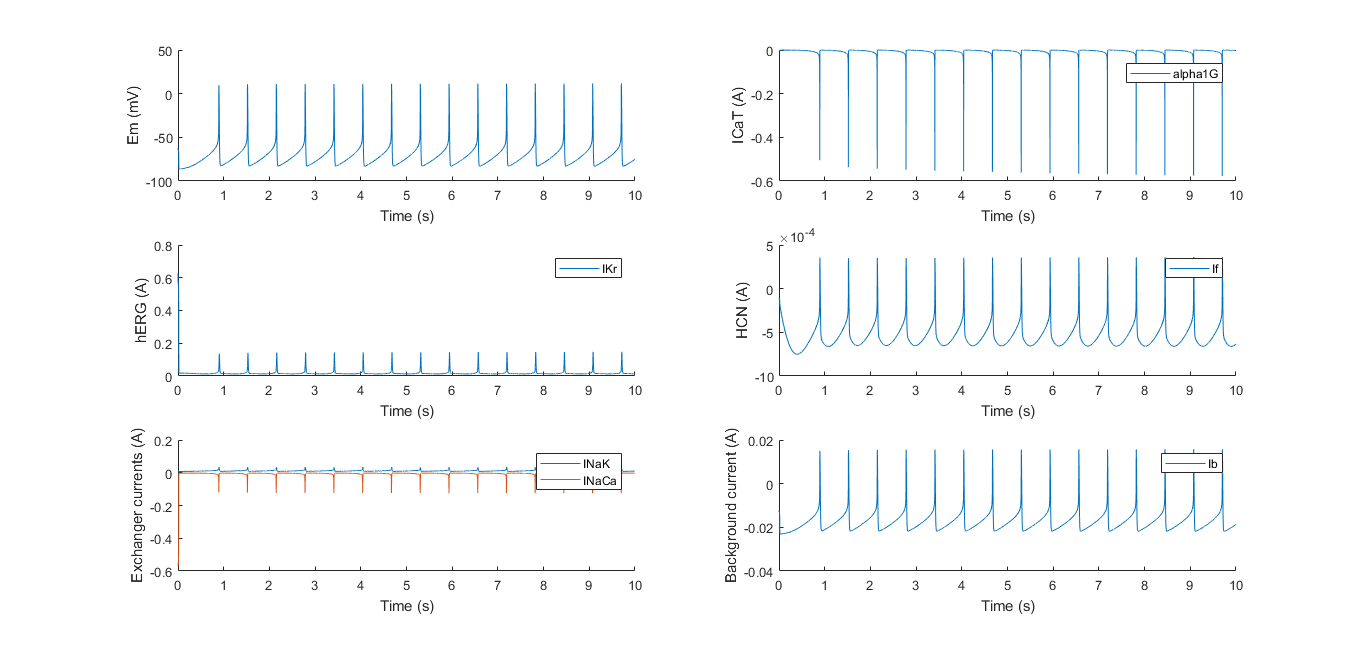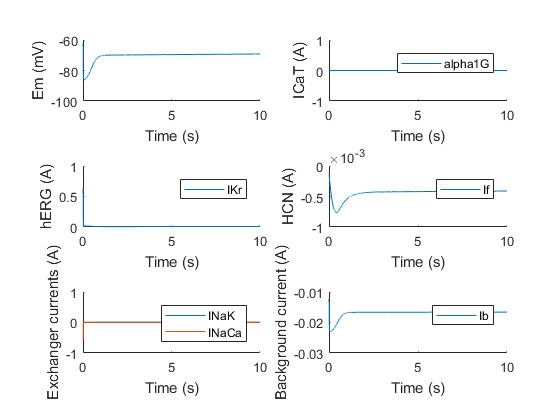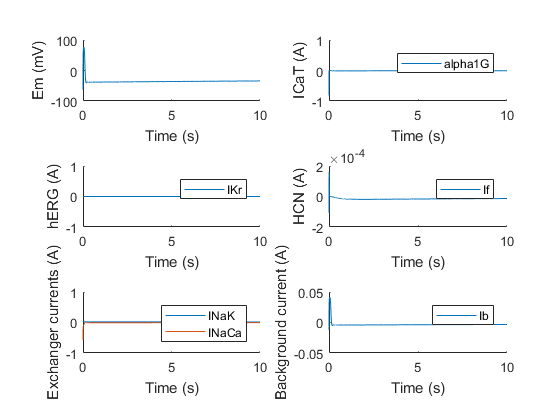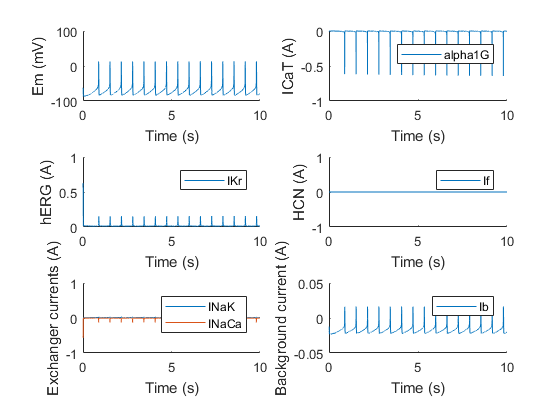# Model

All aspects of the HEKcite project have been inspired by the heart. This is no different for the model. Numerous attempts have been made in the past to mathematically describe the ion currents through the cell membrane of cardiac cells. The efforts include models for different species and different cell types. The purpose of developing these models is to gain insight into the electrical dynamics of the cell in total and the kinetics of its ion channels separately. The practice of modelling these currents dates back to 1952, when Hodgkin and Huxley came up with a model for a giant squid axon that fitted experimental observations quite well. Since then, the search for the perfect model has continued and a lot of researchers have devoted their time to this.

## Introduction into Electrophysiology

To get a proper understanding of how we modelled HEKcite, some basic insights into electrophysiology are needed. More specifically, insights into the mathematical description of electrophysiology. Let us begin by looking closely at the cell membrane. We define the inner side by a, and the outer side by b. In our simplified model of the membrane, we begin by assuming that there is a difference in ion concentrations between the cytosol and the extracellular environment. Because of this concentration gradient, some of these ions will start to diffuse through the membrane. This movement causes an unbalance between the two compartments, resulting in a difference in potential across the membrane: also referred to as $V_m= \phi^a- \phi^b$ For one participating ion species ‘i’, we define $V_{n,i}$ as the Nernst potential. This is the membrane potential whereby ion species ‘i’ is in an electrochemical or thermodynamic equilibrium. In other words, there is no net movement of ‘i’ across the membrane if the Nernst potential is reached. An elegant mathematical description of this potential is: $V_{n,i} = \frac{RT}{z_i F}\ln \frac{c_a^i}{c_b^i}$ , with R the gas constant, T the temperature, zi the valence of the ion, and ci the concentration of the ion. With multiple participating ions all striving to reach their respective Nernst potential, the membrane will eventually reside on a sort of ‘compromise’ potential, called the resting membrane potential Vm.

## Electrical membrane model

Formula: $I_m=G_{Na}(V_r-V_{n,Na})+G_K(V_r-V_{n,K})+G_{Cl}(V_r-V_{n,Cl})$ Equation  gives the electrical current through the membrane as a function of the different electrical conductances of that membrane for each ion (GNa, GK, GCl), the resting membrane potential Vr, and the Nernst potential for each ion (Vn,Na, Vn,K, Vn,Cl). This equation can be interpreted as the sum of electrical currents through the membrane of each different ion. As explained before, every ion has a so-called Nernst potential, at which the ion flux (and thus ion current) through the membrane becomes equal to zero. This means that as long as the Nernst potential of a specific ion is not reached, that ion will cause an ion current that tries to facilitate a change in potential towards that Nernst potential. As exemplified by the potential differences in equation  $(V_r- V_{n,i})$, the effect that is exerted on the potential by an ion gets bigger if the resting potential differs greatly from the Nernst potential. The current is also influenced by the conductivity that the membrane displays for a specific ion. Logically, the more conducting, the more current generated. Because all the Nernst potentials usually divert from the resting membrane potential, they all generate currents (Ii) continuously, resulting in a total current through the membrane Im.

## Hodgkin-Huxley

Up until this point, we have discussed the membrane potential as a static value. In heart cells, however (and more importantly, in our HEKcite cells), the membrane potential is a function of time. To model this, we chose to follow the Hodgkin-Huxley membrane model, which allows the description of the membrane potential in function of time. This is the endpoint we needed for our project. By modelling the membrane potential with different combinations of ion channels, we could select which ion channels were the most ‘electrophysiologically compatible’. Hodgkin-Huxley started by modelling the time courses of conductance of different ions. In the previous section, we assumed conductances as constant values. In a changing environment, this assumption does not hold anymore. Not only is the conductance of ion channels influenced by changing membrane potentials, some of them vary with time itself.

## Different ‘gates’

Hodgkin and Huxley discovered that ion channels can consist of different ‘gates’. Broadly, these ‘gates’ can be classified as potential-dependent, or time-dependent. As an example, we will briefly discuss the sodium channel here. Sodium channels consist of both classes of gates, so are a perfect example to mention here. Firstly, they contain voltage-sensitive gates, which open if a certain cut-off voltage is achieved. Their second class of gates is time-dependent, which, after opening, are inhibited for a period of time. This means that the channels will open when a certain cut-off value is reached, and will then need a certain amount of time to ‘recover’, before they can open again.

## Model Framework

The mathematical model developed for our own HEKcite cells is based on a 2011 paper by Kharche et al., which describes spontaneous action potentials in mouse sinoatrial node cells. The single cell model describes the kinetics of all ion channels found in these cells and the ion fluxes that go through them. This results in more than fourteen different ion channels. The model is said to serve as a way to study arrhythmia and predict the outcome of electrophysiological experiments. To implement this model and use it as a basis for our own, we were very lucky to receive guidance from prof. Eleonora Grandi from University of California, Davis. She was as kind as to provide us with an already existing MATLAB code (Currents,ML1,ML2) for the Kharche model, which served as an excellent starting point for our own model. The first step in producing our HEKcite model, was to omit the currents from the model that would not be of interest to us. The ones that were kept are obviously the funny current If (modeled after HCN4, while HCN2 was used in the HEKcite project), the low voltage activated T-type calcium channel ICaT (α1G or Cav3.1 in both the original model and the project) and the rapid delayed inward rectifier current IKr (mERG in the paper, hERG for the project). Other channels that are endogenous to HEK cells, such as the sodium-potassium pump (INaK) and the sodium calcium (INaCa) exchanger were also included, together with the background currents (Ib). The model basically does two things. First, it calculates the current flowing through a channel in function of the membrane potential. Both the three HEKcite channels and the background currents follow the easy model of current through an electrical circuit, as was explained above. Which means that these currents are of the form: $I = G(V_r-V_{n,i})$ Speaking of circuit theory, a very interesting component finding its surprising way into biology, is the capacitor. In its simplest form it consists of two parallel conducting plates, in between which an insulating medium is placed. If supplied with a voltage charge builds up on both plates, because of the insulator in between. All of this is summarized in this elegant expression: $I(t) = C\frac{dv}{dt}$ If we now return to cell biology, we could say the cell membrane can be seen as a capacitor, with the lipid bilayer being the capacitor’s plates. In the model this translates to the following elegant description of the membrane potential: Secondly, the model updates the concentrations of the contributing ion species, in our case potassium, sodium and calcium. Since these concentrations are very hard to measure experimentally, the Kharche model updates the concentrations in time by means of a simple ionic balance. The HEKcite model was based on this approach.

A big challenge in cardiac modeling is bringing into account the calcium homeostasis in cardiac cells. The calcium handling in the cells is done by the sarcoplasmic reticulum, and is quite intricate. Since HEK cells do not have a sarcoplasmic reticulum, the calcium handling in our model was simplified to calcium exchange being possible over the whole cell surface. The change in ionic concentrations was given the same dynamics as the one for potassium and sodium. For an even better fit with the HEKcite data, several parameter values, such as channel conductances, the size of the cell, and cell (in)activation parameters were adapted. All of this included results in a set of nine differential equations which are solved with MATLAB, given a set of initial conditions provided by the paper.

## Results

The developed model provided the ultimate in silico proof of the HEKcite project. After reducing the amount of currents and adjusting the model to correspond with HEK cells, the model provided the in silico proof that oscillations in HEK cells would be possible with this combination of ion channels.Proof of the importance of each ion channel can be provided by knocking out a channel. If we for instance set the channel conductance of α1G to zero (making its current zero, see above), the result is the following.With the initial condition for the membrane potential set to around -60 mV, we can see activation of the HCN channel, but it clearly does not depolarize enough to activate hERG, which is normally α1G’s job. The same experiment can be done for the other channels. For example, if we knock out hERG, we obtain the following result.Again, the model shows that if hERG is knocked out, oscillations stop, due to the lack of a repolarizing component. Surprisingly however, the model shows that if HCN is knocked out, the oscillations do not necessarily come to a halt, which might be explained due to the properties of α1G and hERG. In nature, almost all the electrophysiological pacemaker use an isoform or combinations of HCN isoforms to drive their pace. This is probably due to stability reasons, as the model also shows that not all combinations of conductances will provide oscillations even without HCN. These conductances are very hard to experimentally measure however, making it difficult to say for sure these combinations exist or are present as is in the cells.Even though the model was based on ionic currents in cardiac cells, the results show that translation to HEK cells can be done, as the modeled result showed good correspondence to experimental data. Ion channel knock-outs in silico can be crucial to fully understand the electrical dynamics of a cell. Comparing the model to experimental data is a useful tool in completely understanding the kinetics of different ion channels.

#### References

1. Kharche, S., Yu, J., Lei, M. and Zhang, H. (2011). A mathematical model of action potentials of mouse sinoatrial node cells with molecular bases. AJP: Heart and Circulatory Physiology, 301(3), pp.H945-H963.
2. Course text: Modellering van de weefselfysiologie, KULeuven, H. Ramon